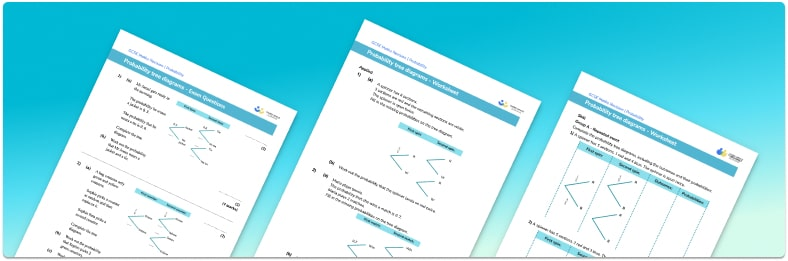# Tree Diagram Worksheet• Section 1 of the tree diagram worksheet contains 12 skills-based tree diagram questions, in 3 groups to support differentiation
• Section 2 contains 4 applied tree diagram questions with a mix of worded problems and deeper problem solving questions
• Section 3 contains 3 foundation and higher level GCSE exam style tree diagram questions
• Answers and a mark scheme for all tree diagram questions are provided
• Questions follow variation theory with plenty of opportunities for students to work independently at their own level
• All questions created by fully qualified expert secondary maths teachers
• Suitable for GCSE maths revision for AQA, OCR and Edexcel exam boards

• This field is for validation purposes and should be left unchanged.

You can unsubscribe at any time (each email we send will contain an easy way to unsubscribe). To find out more about how we use your data, see our privacy policy.

### Tree diagram at a glance

Probability tree diagrams are a way of visually representing a combination of two or more events. Each branch is labelled with an outcome and its probability. Tree diagrams are useful as they help us clearly see all of the possible outcomes. We can find the probability of a combination of events happening by following the appropriate branches of the tree diagram and multiplying the probabilities along those branches.

Tree diagrams can be used both for independent events (e.g. spinning a fair spinner with different colours in each sector) and dependent events (conditional probability), where the probability of an event is affected by a previous event.

Looking forward, students can then progress to additional probability worksheets, for example a Venn diagram worksheet orFor more teaching and learning support on probability our GCSE maths lessons provide step by step support for all GCSE maths concepts.

## Do you have KS4 students who need more focused attention to succeed at GCSE?There will be students in your class who require individual attention to help them succeed in their maths GCSEs. In a class of 30, it’s not always easy to provide.

Help your students feel confident with exam-style questions and the strategies they’ll need to answer them correctly with our dedicated GCSE maths revision programme.

Lessons are selected to provide support where each student needs it most, and specially-trained GCSE maths tutors adapt the pitch and pace of each lesson. This ensures a personalised revision programme that raises grades and boosts confidence.

Find out more